# ACT Math: Triangles Chapter Exam

Exam Instructions:

Choose your answers to the questions and click 'Next' to see the next set of questions. You can skip questions if you would like and come back to them later with the yellow "Go To First Skipped Question" button. When you have completed the practice exam, a green submit button will appear. Click it to see your results. Good luck!

### Page 1

#### Question 3 3. What type of triangle is pictured below?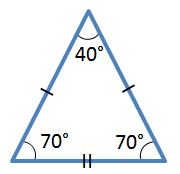#### Question 5 5. Josh claims that these triangles are similar by SSS. Do you agree or disagree with Josh?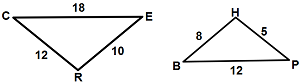### Page 2

#### Question 6 6. What is the area of the pictured triangle?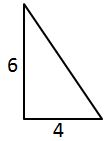#### Question 10 10. In the right triangle pictured below, what is the value of angle x?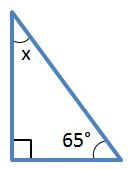### Page 3

#### Question 12 12. Jerry wants to buy a TV online, but the ad for the TV he wants to buy does not say what the screen size is. He knows that TV screens are measured diagonally. This image shows the length and width of the screen, so he decides to use the Pythagorean Theorem to determine the diagonal screen size. What is the screen size of the TV that Jerry wants to buy (to the nearest inch)?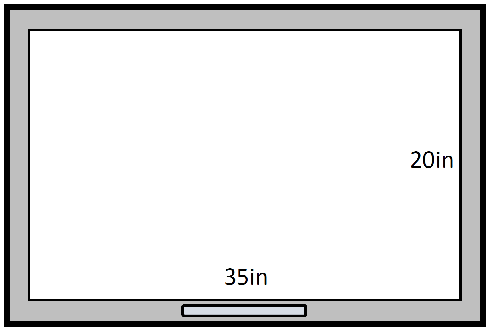#### Question 14 14. What's wrong with this triangle?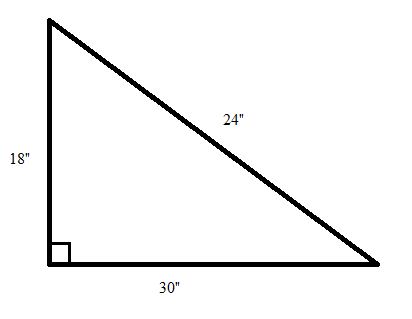#### Question 15 15. Given triangles DAR and KMR. What is the value of y?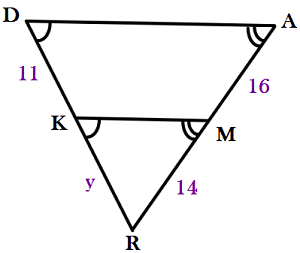### Page 4

#### Question 16 16. Is triangle ABC similar to triangle DEF?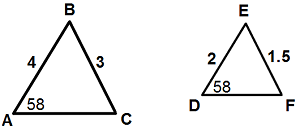#### Question 18 18. Which of the following is true?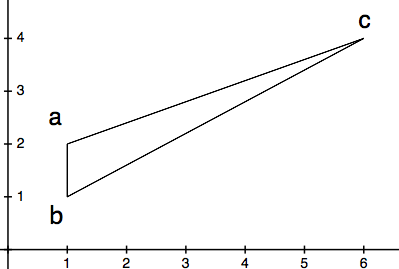#### Question 19 19. What is the area of the pictured rectangle?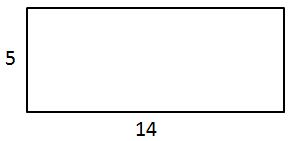### Page 5

#### Question 22 22. In the picture shown below, there are three lines marking each side of the triangle. These lines give the indication that the triangle is: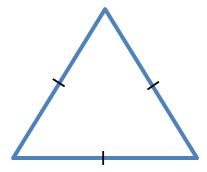#### Question 24 24. Study the given diagram. Find the value of x, the distance between T and E.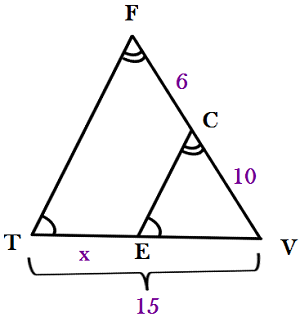### Page 6

#### Question 27 27. The isosceles triangle pictured below shows one angle measuring 40 degrees. What is the measure of angle x?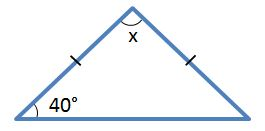#### Question 30 30. Determine if triangle VEZ is similar to triangle MAG.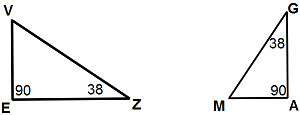#### ACT Math: Triangles Chapter Exam Instructions

Choose your answers to the questions and click 'Next' to see the next set of questions. You can skip questions if you would like and come back to them later with the yellow "Go To First Skipped Question" button. When you have completed the practice exam, a green submit button will appear. Click it to see your results. Good luck!

Support